Study Guide

# Electromagnetism - Electric Fields

## Electric Fields

In the previous unit on electricity and magnetism, we learned how an electric field E exists between two opposite charges q and –q at a distance r from each other. They attract each other, very much like gravity, with a force F given by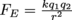.This force is called the Coulomb or the electric force, with units of Newtons like any other force. Let’s also remember an important constant,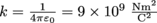.

An electric field is formed by a point charge q, which exerts a force F on a test charge q0. The electric field E is then given by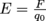, or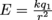and its electric field lines go from the positive to negative direction, since the electric field is a vector quantity just as is the electric force.

Not that we haven’t encountered vectors before with any other forces, but here’re two handy vector refreshers just in case. .

We had fun finding electric fields and forces with point charges. Now it’s time to amp up the volume—literally and figuratively. Memory refreshing is officially over.

Our previous electric force and field calculations were limited to single point charges. What if there were three? Four? More? Then we add the vectors together, of course.

We’ll do an example. Three different charges are held against their will on the corners of a rectangle 4 cm long and 2 cm wide as shown below. What is the net electric field at Point A?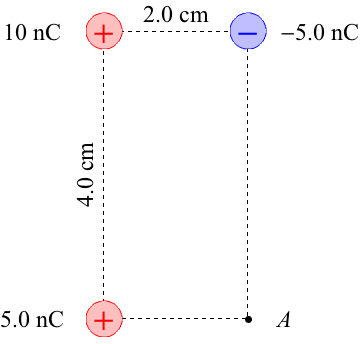What the heck? We know how to find the field from any one point, but not from three. Oh…we find the field from each of the three separately and then add up the x-directions, y-directions and ultimately find the total field by using the Pythagorean Theorem. That makes total sense, right?

The positive and 5 nC charge is straightforward enough with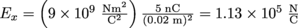.The negative 5 nC one is similar, but with a negative sign (duh) and a different radius, and a different direction: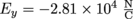.

We can no longer avoid the electric field coming from the 10 nC charge diagonally across from Point A, with a combination of the both directions. We could use the square root of 0.022 + 0.042 to find the numeric value and then split it into Ex and Ey using trigonometry, or we could leave it separate the whole time. Let’s do that. The x distance is 0.02 m, then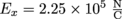, and the y distance is 0.04 so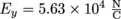.

Next up: add the two x terms together and the two y terms together for a total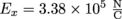and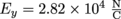.Now is the time to pull out Pythagoras to find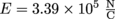, and since the y-component is so much smaller than the x-component, the field points predominantly in the x-direction towards the +5nC charge. We could also find the exact angle from trig (it’s about 5°) but we weren’t asked for direction.

### Electric Fields of Continuous Charge Distributions

Whew! We survived a complicated vector addition of three individual electric fields. Nothing else could be as bad as that, right? Yes, this is a set up. The math is about to get a lot worse, but the good news is that we don’t have to do the math ourselves.

What could possibly be more difficult to calculate? The electric field from a continuum of charges, that’s what. If we have a smear of electric charge spread evenly over some kind of surface, we call this a continuous charge distribution. This means a line, a surface, or the body of a three-dimensional object with a total charge, Q, instead of a single point charge, q, and a more complicated electric field to go with it.

Yeah. We want point charges back too. Before scrambling to hide under the bed in the fetal position, hold on to the idea “we don’t have to do the math ourselves.” We do need to understand the concepts, though.

First concept: a continuous charge distribution has a charge density of charge per distance, area, or volume. Along a charged rod, the charge density is charge per distance, denoted by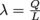.For a charged area, we use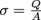.

Next concept: to find the electric field E along a line or surface of uniform charge, we just need to add all the electric field contributions from each place along the rod (or area or volume) with charge on it, leading to different equations for different types of charge distributions. This is done by calculus, but not by us. There is no “us” in calculus today. Try the next level of physics up for that. Phew!

After the calculus, we have various resulting equations for calculating the electric field of different charge distributions and we can still get to electric force by FE = qE for a charge, q, in the presence of any of these electric field conditions below. Ahem, moving on.

### Electric Field within a Charged Spherical Shell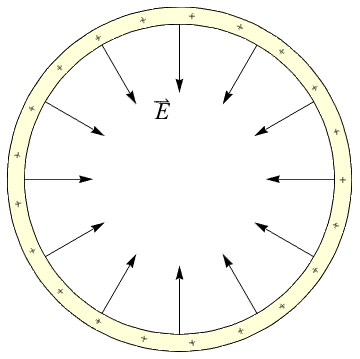As we see from the field lines alone, all the fields added up from all the parts of the spherical shell cancel each other out, leaving absolutely no electric field within a hollow charged sphere. It applies to other shapes as well, and it’s how Faraday cages work. And yes, Michael Faraday invented them to shield whatever is placed inside from exterior electric and current-based magnetic fields.

Note that the electric field within a solid charged sphere does exist: it’s only zero right smack in the center where there’s no enclosed charge at r = 0. The field is proportional to the amount of charge enclosed for r < R where r is some radius within the sphere and R is the outer radius of the charged sphere.

### Electric Field on the Axis of a Rod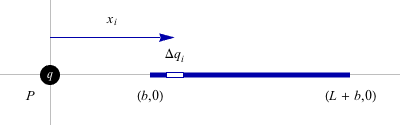For a rod of length L with a continuous charge Q along it, we consider the charge density,contributing to form the electric field at some distance from but in line with the rod, b, is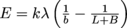### Electric Field on the Axis of a Ring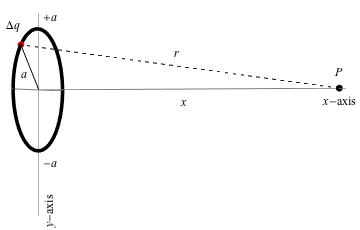For a ring (loop) with a continuous charge on it, the electric field is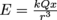where r is the distance from the ring along the center axis of the ring, or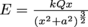### Electric Field on the Perpendicular Bisector of a RodOut in front of the middle of a charged rod, the electric field is different than along the axis. This one is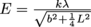. Once again,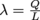.

### Electric Field from a Uniformly Charged Plane

For a charged sheet of material with a uniform charge density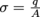,the electric field near the sheet is given by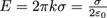. Note that this equation doesn’t have anything to do with distance from the sheet. That’s weird. Why? The electric field points perpendicular to the sheet of metal. It’s uniform!

In real life a sheet of metal doesn’t stretch to infinity, so if we want a uniform (constant) electric field then we place two metal plates near each other with an electric potential between them. They’re like the infinite sheet of uniform charge density, but with two we get the case below, with the same electric field of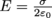.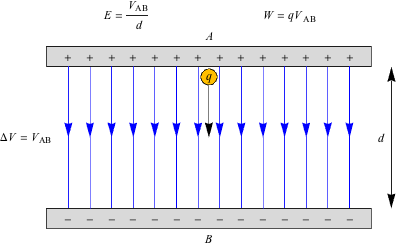### Common Mistakes

For multiple point charges, we have to be very careful to add up their contributing electric fields as vectors, or there’s no hope of arriving at a sensible (and correct) answer.

Continuous charge distributions have their own lurking traps: their shapes. Check and double check that the equation used is the equation which best represents the problem.

### Brain Snack

There are as many equations as there are possible variations in shapes and possible variations between the field on the axis and the field along a bisector. We could calculate the electric field for a disk, sphere, cube, anything. Well, not us. Someone who knows calculus could calculate all those things. And has. Things we look forward to in the future, friends.